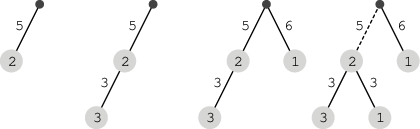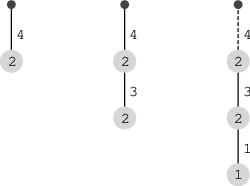hard
Find the maximum number of ropes that can be attached in order, without breaking any of the ropes.
100%
Correctness
100%
Performance
100%

In a room there are N ropes and N weights. Each rope is connected to exactly one weight (at just one end), and each rope has a particular durability − the maximum weight that it can suspend.

There is also a hook, attached to the ceiling. The ropes can be attached to the hook by tying the end without the weight. The ropes can also be attached to other weights; that is, the ropes and weights can be attached to one another in a chain. A rope will break if the sum of weights connected to it, directly or indirectly, is greater than its durability.

We know the order in which we want to attach N ropes. More precisely, we know the parameters of the rope (durability and weight) and the position of each attachment. Durabilities, weights and positions are given in three arrays A, B, C of lengths N. For each I (0 ≤ I < N):

• A[I] is the durability of the I-th rope,
• B[I] is the weight connected to the I-th rope,
• C[I] (such that C[I] < I) is the position to which we attach the I-th rope; if C[I] equals −1 we attach to the hook, otherwise we attach to the weight connected to the C[I]-th rope.

The goal is to find the maximum number of ropes that can be attached in the specified order without breaking any of the ropes.

Write a function:

int solution(int A[], int B[], int C[], int N);

that, given three arrays A, B, C of N integers, returns the maximum number of ropes that can be attached in a given order.

For example, given the following arrays:

A = 5 B = 2 C = -1 A = 3 B = 3 C = 0 A = 6 B = 1 C = -1 A = 3 B = 1 C = 0 A = 3 B = 2 C = 3the function should return 3, as if we attach a fourth rope then one rope will break, because the sum of weights is greater than its durability (2 + 3 + 1 = 6 and 6 > 5).

Given the following arrays:

A = 4 B = 2 C = -1 A = 3 B = 2 C = 0 A = 1 B = 1 C = 1the function should return 2, as if we attach a third rope then one rope will break, because the sum of weights is greater than its durability (2 + 2 + 1 = 5 and 5 > 4).

Write an efficient algorithm for the following assumptions:

• N is an integer within the range [0..100,000];
• each element of array A is an integer within the range [1..1,000,000];
• each element of array B is an integer within the range [1..5,000];
• each element of array C is an integer such that −1 ≤ C[I] < I, for each I (0 ≤ I < N).